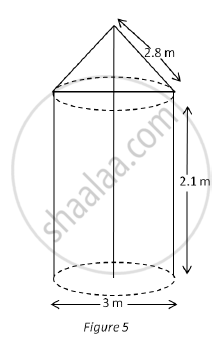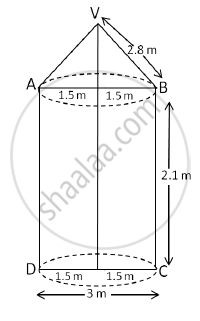# In Fig. 5, a tent is in the shape of a cylinder surmounted by a conical top of same diameter. If the height and diameter of cylindrical part are 2.1 m and 3 m respectively and the slant height of conical part is 2.8 m, - Mathematics

In Fig. 5, a tent is in the shape of a cylinder surmounted by a conical top of same diameter. If the height and diameter of cylindrical part are 2.1 m and 3 m respectively and the slant height of conical part is 2.8 m, find the cost of canvas needed to make the tent if the canvas is available at the rate of Rs. 500/sq. metre ( "Use "pi=22/7)#### Solution

For conical portion, we have

r = 1.5 m and l = 2.8 m

∴ S1 = Curved surface area of conical portion

∴ S1 = πrl

= π × 1.5 × 2.8

= 4.2π m2

For cylindrical portion, we have

r = 1.5 m and h = 2.1 m

∴ S2 = Curved surface area of cylindrical portion

∴ S2 = 2πrh

= 2 × π × 1.5 × 2.1

= 6.3π m2

Area of canvas used for making the tent =S1+S2

=4.2π + 6.3π

=10.5π

=10.5 x 22/7

= 33 m2

Total cost of the canvas at the rate of Rs. 500 per m2 Rs.(500 x 33)=Rs.16500Concept: Concept of Surface Area, Volume, and Capacity
Is there an error in this question or solution?